Courses

# Test: Refraction By Spherical Surfaces

## 5 Questions MCQ Test Physics For JEE | Test: Refraction By Spherical Surfaces

Description
This mock test of Test: Refraction By Spherical Surfaces for JEE helps you for every JEE entrance exam. This contains 5 Multiple Choice Questions for JEE Test: Refraction By Spherical Surfaces (mcq) to study with solutions a complete question bank. The solved questions answers in this Test: Refraction By Spherical Surfaces quiz give you a good mix of easy questions and tough questions. JEE students definitely take this Test: Refraction By Spherical Surfaces exercise for a better result in the exam. You can find other Test: Refraction By Spherical Surfaces extra questions, long questions & short questions for JEE on EduRev as well by searching above.
QUESTION: 1

### A ray of light going from denser to rarer medium suffers refraction at a concave surface. Which of the following relations is correct?

Solution:

Solution :

The correct option is Option A.

Laws of refraction;-

The incident ray,the refracted ray and the normal to the refracting surface at the point of incidence lie in the same plane.

For a given pair of media and for a given colour of light the ration between the sine of angle of incidence to the sine of refraction is a constant.This constant is known as refractive index of the second medium with respect to the first medium.

When a ray of light passes through a glass slab, ∠i,∠r and the normal all lie in the same plane.

When a ray of light passes from one medium to another, here from air to glass or glass to air, the ratio sini / sinr = constant.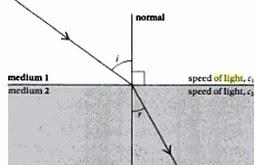QUESTION: 2

### For a plane surface, the formula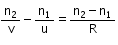becomes

Solution:

For plane mirror R=infinity
so. (n2-n1)/R=0
therefore, n2/V=n1/U

QUESTION: 3

### Consider a curved surface between two different media with refractive indices n1 = 1 and n2 = 2. The relation between radius of curvature, image distance and object distance is given by

Solution:

The relation between radius of curvature, image distance and object distance is given by
n2/v-n1/u=n2-n1
un2-vn2/vu=n2-n1/R
R=vu(n2-n2)/un2-vn2
=uv(2)/u(2)-v(1)
=[uv/2u-v)

QUESTION: 4

In the adjoining figure, SS is a spherical surface separating two media of refractive indices n1 and n2 where n1 > n2. C is the centre of curvature of the spherical surface. An observer, keeping his eye beyond C in the medium of refractive index n2 views the refracted image of an object AB placed as shown in the medium of refractive index n1. The image will be: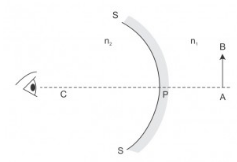Solution: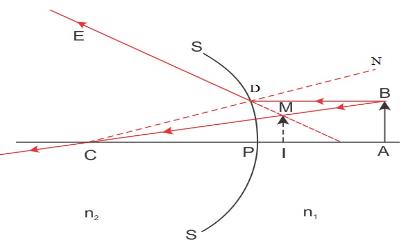The image will be virtual, upright and diminished.

QUESTION: 5

A thick plano convex lens made of crown glass (refractive index 1.5) has a thickness of 3cm at its centre. The radius of curvature of its curved face is 5cm. An ink mark made at the centre of its plane face, when viewed normally through the curved face, appears to be at a distance ‘x’ from the curved face. Then, x is equal to: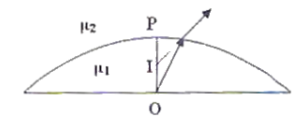Solution:

A thick Plano convex lens made of crown glass (refractive index 1.5) has a thickness of 3cm at its centre. The radius of curvature of its curved face is 5cm. An ink mark made at the centre of its plane face, when viewed normally through the curved face, appears to be at a distance ‘x’ from the curved face, and we have to determine the value of ' x'
According to the picture, the ray of light gets refracted at the interface between the air and the lens from the object 'p' and 'I' is the refracted image of 'p'
object distance 'u'= BO
Image distance 'v'/'x' = BI
we know
n2/v - n1/u = (n2- n1) / R
or 1/v - 1.5/(-3 )= (1-1.5)/ (-5) [where n2= 1, n1= 1.5 ,u= -3 ,R= -5]
so, 1/v = -6/15
or, v = -2.5
so, x is equal to 2.5 cm